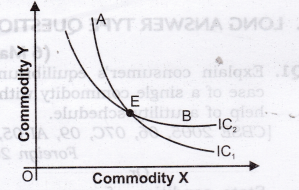# Chapter 2 – Consumer Equilibrium Questions and Answers: NCERT Solutions for Class 12 Micro Economics

Class 12 Introduction to Economics NCERT book solutions for Chapter 2 -Consumer Equilibrium Questions and Answers.## Questions 5, 6 and 7 are related to question 4, Question 5. How does the budget line change if the consumer’s income increases to ?40 but the prices remain unchanged?

### Answer: If consumer’s income increases to Rs 40, the consumer can buy more pieces/quantities of both the goods X and Y. There will be parallel rightward shift in the budget line AB to A1B1.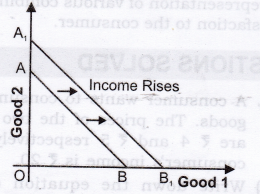## Question 6. How does the budget line change if the price of good 2 decreases by a rupee but the price of good 1 and the consumer’s income remain unchanged?

### Answer: If price of good 2 (shown on y-axis) decreases, consumer can buy more pieces /quantity of good 2. The budget line AB will pivot at B and rotate upwards to A1 B.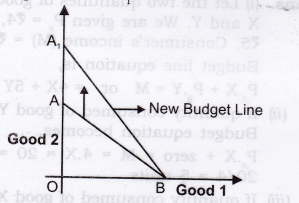## Question 1. Explain with diagram, the relationship between TU and MU.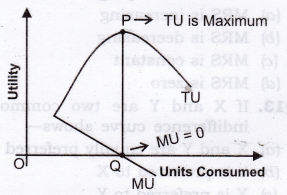## Question 5. Explain the law of diminishing marginal utility with the help of a total utility schedule.

### Answer: The law states that marginal utility derived from the consumption of a commodity declines as more units of that commodity are consumed.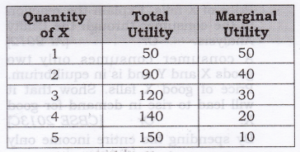## Question 6. Derive MU Schedule from TU Schedule.

###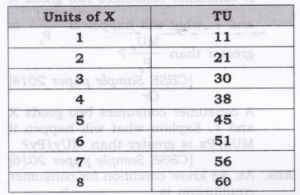Answer: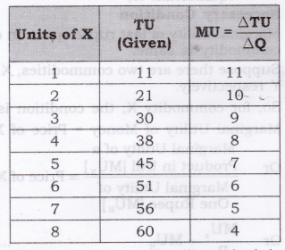## Question 7. A person’s marginal utility schedule is given below. Derive their total utility schedule.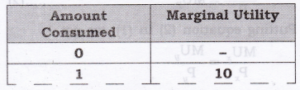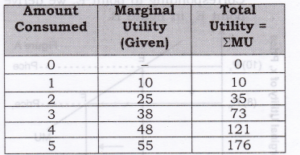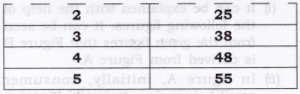## Question 8. Calculate: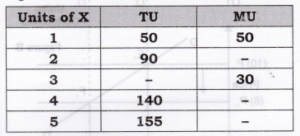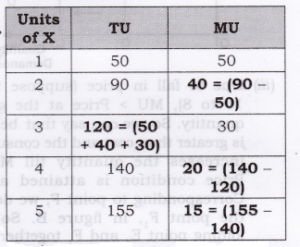## Question 9. Derive the inverse relation between price of the good and its demand from single commodity equilibrium condition “marginal utility = price”.

### Answer: As we know a consumer purchases a good up to the point where marginal utility of the good becomes equal to the price of that good. MU = Price 1. Figure B is derived from Figure A. 2. In figure A, initially, consumer equilibrium is attained at point E, where let MU (10) = Price (10). Corresponding to point E, we derive point E1 in figure B.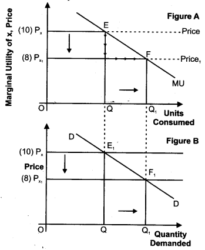## Question 10. A consumer consumes only two goods X and Y. At a consumption level of these two goods, he finds that the ratio of marginal utility to price in case of X is higher than that in case of Y. Explain the reaction of the consumer. Or A consumer consumes only two goods X and Y and is in equilibrium. Price of X falls. Explain the reaction of the consumer through the Utility Analysis. Or A consumer consumes only two goods X and Y and is in equilibrium. Price of good X falls. Show that it will lead to rise in demand for good X. Or By spending his entire income only on two goods X and Y a consumer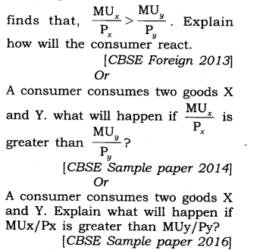### Answer: As, we know condition for consumer equilibrium is, Necessary Condition Marginal utility of last rupee spent on each commodity is same. Suppose there are two commodities, X and Y respectively. So, for commodity X, the condition is, Marginal Utility of Money = Price of X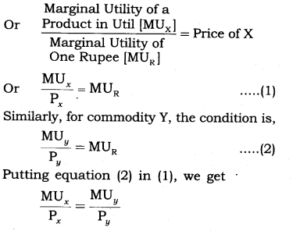## Question 11. A consumer consumes only two goods X and Y. At a certain consumption level of these goods, he finds that the ratio of marginal utility to price in case of X is lower than that in case of Y. Explain the reaction of the consumer. Or By spending his entire income only on two goods X and Y a consumer finds that,MUxpx Or A consumer consumes only two goods X and Y and is in equilibrium. Show that when the price of good X rises, the consumer buys less of good X. Use utility analysis.

### Answer: As, we know condition for consumer equilibrium is, Necessary Condition Marginal utility of last rupee spend on each commodity is same. Suppose there are two commodities, X and Y respectively. So, for commodity X, the condition is, Marginal Utility of Money = Price of X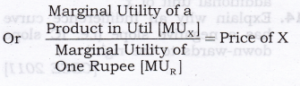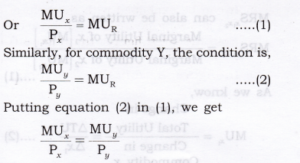## Question 12. Explain the meaning of diminishing marginal rate of substitution with the help of a numerical example.

### Answer: MRS is the rate at which a consumer is willing to sacrifice one commodity for an extra unit of another commodity without affecting his total satisfaction.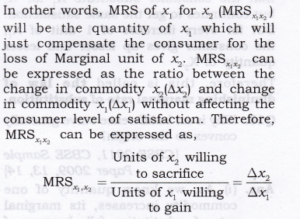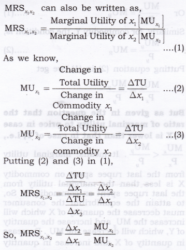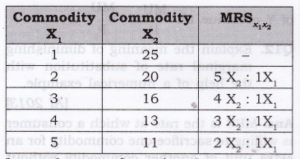## Question 13. Why is an Indifference curve generally , convex to the origin?

### Answer: 1. As, we know quantity of one commodity increases, its marginal rate of substitution falls because of law of diminishing marginal utility. Marginal rate of substitution is a slope of Indifference curve and whenever slope [MRS] decreases it makes the curve convex to the point of origin.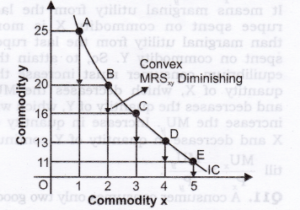## Question 14. Explain why an Indifference curve has a negative slope

### Answer: Every IC is based on the assumption that various combinations of two commodities gives equal satisfaction to a consumer. In order to remain at the same level of satisfaction, the consumer will have to reduce the consumption of one commodity if he wants to increase the consumption of another commodity.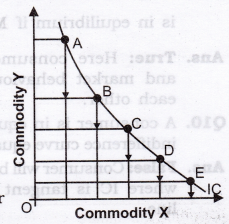## Question 15. Why do Indifference curves not intersect each other?

### Answer: 1. Two IC’s cannot intersect each other. This property is proved by Contradict Method. First we assume that they intersect each other and then show that this assumption leads to an absurd conclusion. Let us assume that IC1 intersects IC2 at point E shown in the figure given here.